Keyah Math Module 12, Level 4

Mathematical Content: Exponential and logarithmic functions, algebraic operations, graphs

# Finding the Age of the Earth: Level 4

Solution to Question 3.

Solve the equation

0.0205 = eλt – 1,

with λ = decay constant for Rb-Sr = 1.42 x10-5, for t.

We present two solutions, one is algebraic, the other is graphic using the plot-solve applet.

Algebraic solution.

Solve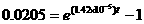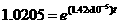Take the natural logarithm of both sides and then solve for t,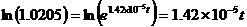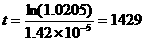.

The gabbro is, approximately, 1,429 million years old.

Graphical solution.

To solve the equation, graph each side and determine the point of intersection.

(The graph shown below is copied from the Earth Math or Kéyah Math website Plot/Solve applet.)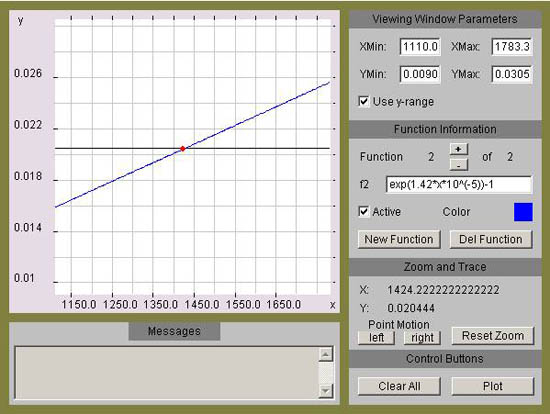The gabbro is, approximately, 1,429 million years old.

This material is based upon work supported by the National Science Foundation under Grant GEO-0355224. Any opinions, findings, and conclusions or recommendations expressed in this material are those of the authors and do not necessarily reflect the views of the National Science Foundation.